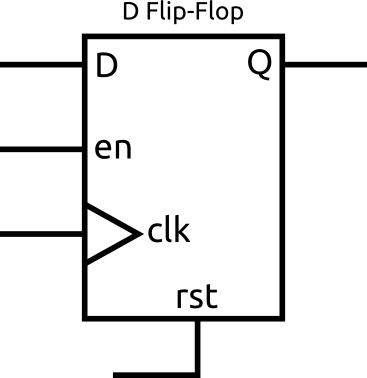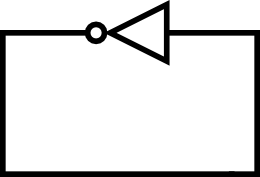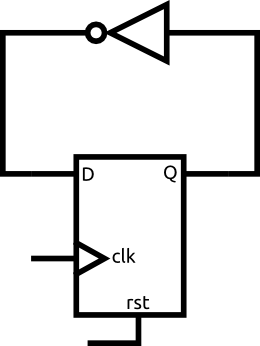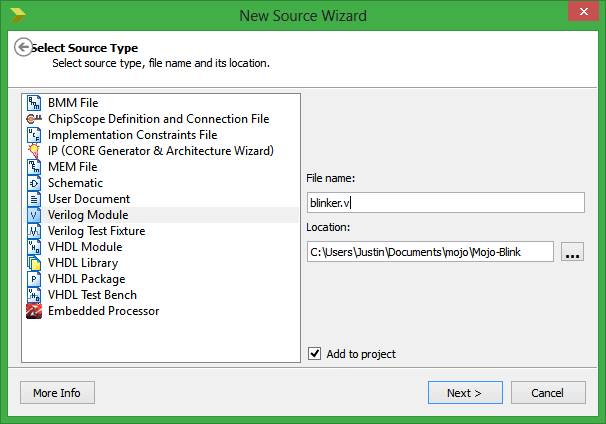# Synchronous Logic

Synchronous logic if a fundamental building block for just about any digital design. It allows you to create much more complex systems that accomplish something over a series of steps. It also gives you the ability to save states or other information. In this tutorial you will be introduced to the D flip flop and how you can use these to make an LED blink.

### The Problem

In our last tutorial we simply wired a LED to a button. Whenever you pressed the button the LED would turn on. Our design had no concept of time. The goal for this project is to blink an LED. That means we have to create a circuit that can turn itself on and off automatically after a regular interval of time has passed. For this we need the flip flop.

### Clocks

Before I can explain what exactly a flip flop does you need to understand what a clock is.

A clock is just a signal that toggles between 0 and 1 over and over again. It looks something like this.The important thing is the rate at which it toggles. The clock on the Mojo is a 50MHz clock. That means that it toggles 50 million times per second!

The clock has two edges, the rising edge and the falling edge. I drew the rising edges with little arrows. The rising edge is usually the important one.

### D Flip-Flops

This is one of the most important circuit elements you will be using. Lucky for you, it is also fairly straight forward how they work. Take a look at the symbol for the flip flop below.This image shows all the signals that a flip flop could have, but in practice, the only required signals are D, Q, and clk. For now, forget about rst and en.

So what exactly does this thing do? All it does is copy the signal at D to Q whenever there is a rising edge on the clk input. That means Q will keep it's value between rising edges of the clock. Since the flip flop remembers what the input was at D, it is actually one of the most basic memory elements.

### Loops

Lets take a look at the following example.What will this circuit do? If the input to the gate is 1, then it's output is 0. However, the output is the input so the output must be 1, but then the input is 1 so the output must be 0? If we assume that the signal can only be 0 or 1 it seems like it would toggle between 0 and 1 infinitely fast. In practice, remember that signals are actually represented by voltages, it may oscillate or it may settle to somewhere in the middle. This is of course something we don't want! Designing combinational circuits with feedback can be very tricky to make sure something like this doesn't happen and that the circuit will work how you expect it to. That is why we don't! Instead, we use a circuit like this one.What will this circuit do? Well, for now lets just assume that Q is 0. That means that D is 1 (because it went through the not gate). On the next rising edge of the clock Q will copy what D is, so Q becomes 1. Once Q becomes 1, D becomes 0! You can follow the pattern to realize that every time there is a rising edge on the clock the output of the flip flop toggles.

What about the initial condition though? If we just built this circuit how do we know if Q is 0 or 1? The truth is that we don't and in some cases it may be 1 while others it may be 0. That is where the rst signal comes in. This signal is used to reset the flip flop to a known state. In FPGAs this signal is generally very flexible and allows you to reset the flip flop to a 1 or 0 when the signal is high or low (your choice, not both). I personally like to use an active high reset. That means when the rst signal is 1, the flip flop is held in reset.

There are cases where you don't care what the inital value of the flip flop is, in those cases you don't need to, and shouldn't, use a reset.

Since the only signal left is en, I'll cover it now just for completeness. There are times when you want the flip flop to ignore the rising edges on the clock and to preserve the contents of Q. That is when you use the enable signal. When the en signal is 1, the flip flop operates normally. When it is 0 the contents of Q won't change on the rising edges of the clock. If you see a flip flop without an en signal it is just assumed that the flip flop is always enabled.

### Creating the blinker module

We are going to modify the code from the previous tutorial to create the blinker, so make sure you've downloaded the base project

Open up the project in ISE. We now are going to add a new module which will be our blinker. On the left in the Hierarchy section right click on mojo_top and choose New Source...Select Verilog Module and enter blinker.v as the name. Click Next then Next then Finish.

The file should open in the editor. Replace the existing code with the following.

```module blinker(
input clk,
input rst,
output blink
);

reg [24:0] counter_d, counter_q;

assign blink = counter_q;

always @(counter_q) begin
counter_d = counter_q + 1'b1;
end

always @(posedge clk) begin
if (rst) begin
counter_q <= 25'b0;
end else begin
counter_q <= counter_d;
end
end

endmodule```

Just like the last tutorial lets break down the code to understand what is going one.

### The reg

`reg [24:0] counter_d, counter_q;`

This is the first new piece of code. Reg stands for register, and is a bit different than a wire. Flip flops are sometimes called registers. However, a confusing thing in Verilog is that a reg does not necessarily become a flip flop and they can be used in ways similar to wires.

The wire type is used simply to connect to things. A wire can only have one value, in other words it can only be connected to one thing and it will always be connected to that thing. A reg can actually drive a signal. These points are very subtle and the line between them can get blurry in some cases.

The only really important thing to know is that a reg can be assigned a value in an always block while wires can not (they can be read though). Any time you use always blocks you will be using reg's. If you are just connecting two signals you will be using wires.

Here we are declaring two 25 bit wide reg's. We are going to use these signals to create a 25 bit wide flip flop (made up of 25 1 bit flip flops). The suffixes _d and _q corresponding to the flip flop signals d and q from before. It is good practice to use this nameing convention.

### Combinational always blocks

```  always @(counter_q) begin
counter_d = counter_q + 1'b1;
end```

This is our first always block. Always blocks can kinda be thought of as functions that run under certain conditions. Those conditions are specified in the sensitivity list. The sensitivity list in this case is just counter_q. This is because the values this always block produces will only change when counter_q changes.

Since this is out combinational always block, we could have used @(*) for the sensitivity list. This says that this always block will be run whenever anything changes. The only reason not to do this is for simulations. In a simulation the always blocks are run basically as functions and limiting the sensitivity list to only the signals of interest will help reduce the time needed to run the simulation. For synthesis (the actual FPGA design) it doesn't make any difference since it will be in hardware and will just exist. Many times I just use @(*) for my sensitivity lists though.

The line inside the block is very important. This line says that the d signal to the flip flop will get the q value plus 1. This is very similar to our example with the flip flop and the not gate. We are making a loop.

### Synchronous always blocks

```  always @(posedge clk) begin
if (rst) begin
counter_q <= 25'b0;
end else begin
counter_q <= counter_d;
end
end```

This block is a bit more interesting. The sensitivity list for synchronous always blocks is very important. The list uses the keyword posedge. This means that the block is only sensitive to the positive edge of the signal, in other words, a transition from a 0 to a 1. This is exactly what a flip flop's clock input is sensitive to.

Verilog has no direct way to create flip flops. Instead, you have to use a template, like this block, for the tools to realize that is what you wanted. This block basically just describes the function of a flip flop that has input counter_d, output counter_q, clock clk, and reset rst

The if statement in the block is used to reset the flip flop when rst is 1. When rst is 0, the flip flop will copy the value of counter_d to counter_q at the positive edge of clk

### The counter

Let's look at what this module will actually do. Right after the rst signal goes low, counter_q will be 0. That means that counter_d will be 1, since our combiational block assigns it counter_q plus 1.

At the next positive edge of clkcounter_q will be assigned the value of counter_d, or 1. Once counter_q is 1, counter_d must be 2! You should be able to see what will continue to happen. Each clock cycle counter_q will increase by 1. But what happens when counter_q is 25'b1111111111111111111111111 (the max value)?

Since we are adding a 1 bit number to a 25 bit number, the result can be up to 26 bits, but since we are storing it into a 25 bit reg we lose the last bit. That means that when counter_q is the max value, counter_d is 0 and the process starts all over again.

Our counter will continuously count from 0 to 33554431 (2^25 - 1).

How do we make an LED blink from this counter? It's simple once  you realize that for half the time the most significant bit is 1 and the other half of the time it's 0. This is because if counter_q is less than 16777216 (2^24), then the 24th but must be 0 and when it is equal or greater it must be 1. That means we can just connect the LED to the most significant bit of our counter! If you need to convince yourself this is true try writing out the binary values from 0-7 and look at how the MSB (most significant bit) changes.

How fast will the LED blink though? We know that the clock frequency is 50MHz, or 50,000,000 clock cycles per second. Since our counter takes 2^25 cycles to overflow we can calculate the blinker time, 2^25 / 50,000,000 or about 0.67 seconds. The LED will turn on and off every 0.67 seconds. If you wanted to make that time longer, you can just make the counter 26 bits long and the time will double to 1.34 seconds. If you wanted to make it blink faster you can make the counter shorter.

### Non-blocking assignments

You may have noticed that in our combinational block we used = to assign counter_d a value, but in the synchronous block we used <= to assign the value. The <= operator is used for non-blocking assignments. All that means is all the values assigned with <= get assigned immediately in parallel.

When you use just =, which is used for blocking assignments, that line must finish before the next one can happen.

This isn't too important now, just know that <= is generally used in the synchronous block while = is used in the combinational block.

### Instantiating a module

Now we have to instantiate our module from the top level of our design. Open up mojo_top and edit it so it looks like the following.

```module mojo_top(
// 50MHz clock input
input clk,
// Input from reset button (active low)
input rst_n,
// cclk input from AVR, high when AVR is ready
input cclk,
// Outputs to the 8 onboard LEDs
output[7:0]led,
// AVR SPI connections
output spi_miso,
input spi_ss,
input spi_mosi,
input spi_sck,
// AVR ADC channel select
output [3:0] spi_channel,
// Serial connections
input avr_tx, // AVR Tx => FPGA Rx
output avr_rx, // AVR Rx => FPGA Tx
input avr_rx_busy // AVR Rx buffer full
);

wire rst = ~rst_n; // make reset active high

// these signals should be high-z when not used
assign spi_miso = 1'bz;
assign avr_rx = 1'bz;
assign spi_channel = 4'bzzzz;

assign led[7:1] = 7'b0;

blinker awesome_blinker (
.clk(clk),
.rst(rst),
.blink(led)
);

endmodule```

You can see where our blinker is being instantiated at line 24. The first line tells what module we are instantiating (blinker) and what we want to name this instance of it (awesome_blinker). The rest of the code is used to connect the inputs and outputs of our module. The module signal name is the first part after the . and the signal to connect it to is in the ().

In this case we just connect the clock and reset lines and the output to one of the LEDs.

You should now be able to synthesize your design and load it onto the Mojo to see an LED blink about 2x per second.